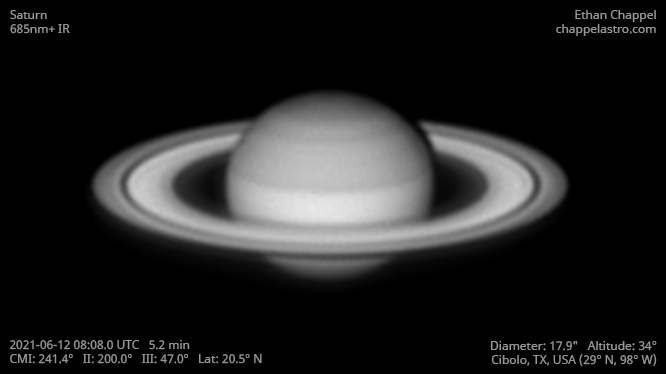# Saturn 2021-06-12 08:08 UTC

CM1: 241.40°

CM2: 200.00°

CM3: 47.00°

CLat: +20.50°

Description

Good seeing conditions, but clouds rolled in just as I started recording. This was the first night with my new Sky-Watcher EQ6-R Pro. While my primary use for this mount will be for deep sky imaging, it will also let me use the EdgeHD 11 in times I would have used the EdgeHD 8 for planetary imaging.

Equipment

Celestron EdgeHD 11

Logs
```FireCapture v2.7 04 BETA Settings
------------------------------------
Observer=Ethan Chappel
Location=Cibolo
Scope=Celestron C8 EdgeHD
Camera=ZWO ASI462MC
Filter=IR685
Profile=Saturn
Diameter=17.80"
Magnitude=0.45
CMI=240.9° CMIII=46.5°  (during mid of capture)
FocalLength=2800mm (F/13)
Resolution=0.21"
Filename=s2021-06-12_08-07-17_ir685_ec.ser
Date=2021_06_12
Start=08_06_32.270
Mid=08_07_17.753
End=08_08_03.237
Start(UT)=08_06_32.270
Mid(UT)=08_07_17.753
End(UT)=08_08_03.237
Duration=90.967s
Date_format=yyyy_MM_dd
Time_format=HH_mm_ss
LT=UT
Frames captured=2548
File type=SER
Binning=1x1
ROI=400x562
ROI(Offset)=0x0
FPS (avg.)=28
Shutter=33.62ms
Gain=409 (68%)
AutoExposure=off
AutoGain=off
Brightness=1
Gamma=50
HighSpeed=off
USBTraffic=100
WBlue=95
WRed=52
Histogramm(min)=0
Histogramm(max)=191
Histogramm=74%
Noise(avg.deviation)=1.50
Limit=180 Seconds
Sensor temperature=81.7°F
Focuser position=1830
FireCapture v2.7 04 BETA Settings
------------------------------------
Observer=Ethan Chappel
Location=Cibolo
Scope=Celestron C8 EdgeHD
Camera=ZWO ASI462MC
Filter=IR685
Profile=Saturn
Diameter=17.80"
Magnitude=0.45
CMI=242.0° CMIII=47.6°  (during mid of capture)
FocalLength=2500mm (F/12)
Resolution=0.24"
Filename=s2021-06-12_08-09-05_ir685_ec.ser
Date=2021_06_12
Start=08_08_42.155
Mid=08_09_05.329
End=08_09_28.503
Start(UT)=08_08_42.155
Mid(UT)=08_09_05.329
End(UT)=08_09_28.503
Duration=46.348s
Date_format=yyyy_MM_dd
Time_format=HH_mm_ss
LT=UT
Frames captured=1576
File type=SER
Binning=1x1
ROI=400x562
ROI(Offset)=0x0
FPS (avg.)=34
Shutter=33.62ms
Gain=361 (60%)
AutoExposure=off
AutoGain=off
Brightness=1
Gamma=50
HighSpeed=off
USBTraffic=100
WBlue=95
WRed=52
Histogramm(min)=0
Histogramm(max)=157
Histogramm=61%
Noise(avg.deviation)=0.86
Limit=180 Seconds
Sensor temperature=84.2°F
Focuser position=1830
```